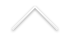Data: 03/05/2018
Hora: 13:00
Local: Sala C119
Palestrante: Marcos Cossarini (IMPA)

Título: Discrete geometry of surfaces towards the filling area conjecture

Resumo: In 1983, Gromov conjectured that the hemisphere has smallest area among Riemannian surfaces that fill isometrically a circle of given length. We discuss the history of the problem and present a discrete version, where a cycle graph of length 2n is filled isometrically by a combinatorial square-celled surface with the least possible number of cells, conjectured to be n(n-1)/2. The discrete problem is equivalent to Gromov's filling area conjecture, as extended by Ivanov to admit filling surfaces with self-reverse Finsler metrics, because every such continuous metric can be replaced by a square-celling whose lengths and areas approximate the original values as precisely as desired (quasi-isometrically with arbitrarily good additive and multiplicative constants).